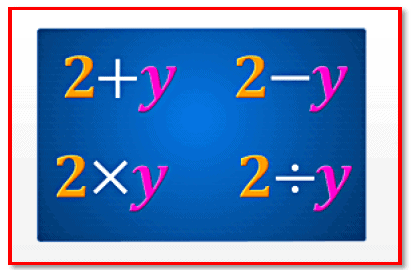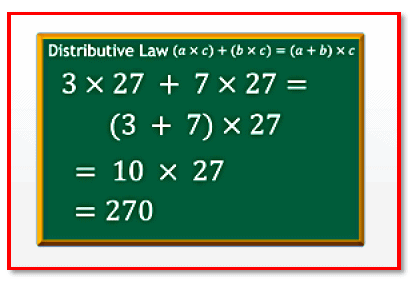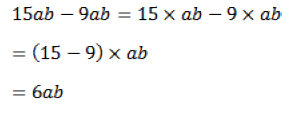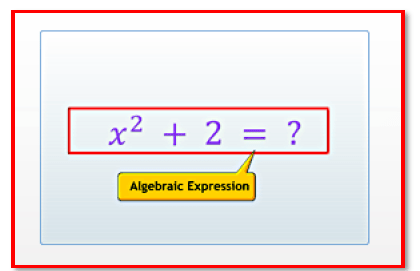Courses

# Chapter Notes - Algebraic Expressions Class 7 Notes | EduRev

## Mathematics (Maths) Class 7

Created by: Praveen Kumar

## Class 7 : Chapter Notes - Algebraic Expressions Class 7 Notes | EduRev

The document Chapter Notes - Algebraic Expressions Class 7 Notes | EduRev is a part of the Class 7 Course Mathematics (Maths) Class 7.
All you need of Class 7 at this link: Class 7

12. Algebraic Expressions

Understanding Algebraic ExpressionsExpressions that contain only constants are called numeric or arithmetic expressions.

Expressions that contain only constants are called numeric or arithmetic expressions. Expressions that contain constants and variables, or just variables, are called algebraic expressions.

While writing algebraic expressions, we do not write the sign of multiplication. An algebraic expression containing only variables also has the constant 1 associated with it. The parts of an algebraic expression joined together by plus (+) signs are called its terms. A term that contains variables is called a variable term.

A term that contains only a number is called a constant term. The constants and the variables whose product makes a term of an algebraic expression, are called the factors of the term. The factors of a constant term in an algebraic expression are not considered. The numerical factor of a variable term is called its coefficient. The variable factors of a term are called its algebraic factors.

Terms that have different algebraic factors are called unlike terms. Terms that have the same algebraic factors are called like terms. Algebraic expressions that contain only one term are called monomials. Algebraic expressions that contain only two unlike terms are called binomials. Algebraic expressions that contain only three unlike terms are called trinomials. All algebraic expressions that have one or more terms are called polynomials. Therefore, binomials and trinomials are also polynomials.

Page 49

Operations on Algebraic ExpressionsWe can perform arithmetic operations on algebraic expressions. To add the like terms in an algebraic expression...

We can perform arithmetic operations on algebraic expressions. To add the like terms in an algebraic expression, multiply the sum of their coefficients with their common algebraic factors.To subtract the like terms in an algebraic expression, multiply the difference of their coefficients with their common algebraic factors. For example, let us subtract 9ab from .15abThus, to add or subtract algebraic expressions, rearrange the terms in the sum of the given algebraic expressions, so that their like terms and constants are grouped together. While rearranging the terms, move them with the correct '+' or '-'sign before them.
Let us see this example.

Add 4x + 12 and 8x - 9

We know that, the terms 4x and 8x are like terms and 12 and -9 are items.

Thererfore,

= (4 + 8) x + 3

= 12x + 3

Unlike terms remain unchanged in the sum or difference of algebraic expressions.

Page 50

Application of Algebraic ExpressionsAlgebraic expressions can be used to represent number patterns.

Algebraic expressions can be used to represent number patterns. In the following example, we can find the relation between number of cones and the number of ice-cream scoops.

 Number of cones  (n) Number of ice - cream scoops (2n) 1 2 2 4 3 6 4 8 15 2 x 15

Thus, we can find the value of an algebraic expression if the values of all the variables in the expression are known.

In a similar way, we can write formulas for the perimeter and area for different geometrical figures using simple, easy-to-remember algebraic expressions

• If s represents the side of a square, then its:
o Perimeter is 4s
o Area is s2

•  If l represents the length and b represents the breadth of a rectangle, then its:Perimeter is 2(l + b) Area is  l x b

Offer running on EduRev: Apply code STAYHOME200 to get INR 200 off on our premium plan EduRev Infinity!

,

,

,

,

,

,

,

,

,

,

,

,

,

,

,

,

,

,

,

,

,

;